Reach Us+441474556909
Design of a Model-following Controller Using a Decoupling Active Disturbance Rejection Control Method | OMICS International
Journal of Electrical & Electronic Systems
All submissions of the EM system will be redirected to Online Manuscript Submission System. Authors are requested to submit articles directly to Online Manuscript Submission System of respective journal.

# Design of a Model-following Controller Using a Decoupling Active Disturbance Rejection Control Method

Inoue S1* and Ishida Y2

1Graduate School of Science and Technology, Meiji University, Japan

2School of Science and Technology, Meiji University, Japan

*Corresponding Author:
Inoue S
Graduate School of Science and Technology
Meiji University, 1-1-1 Higashi-mita, Tama-ku
Kawasaki-shi, Kanagawa, 214– 8571, Japan
Tel: +81 3-3296-4545
E-mail: [email protected]

Received Date: November 05, 2015; Accepted Date: February 05, 2016; Published Date: February 12, 2016

Citation: Inoue S, Ishida Y (2016) Design of a Model-following Controller Using a Decoupling Active Disturbance Rejection Control Method. J Electr Electron Syst 5:174. doi:10.4172/2332-0796.1000174

Copyright: © 2016 Inoue S, et al. This is an open-access article distributed under the terms of the Creative Commons Attribution License, which permits unrestricted use, distribution, and reproduction in any medium, provided the original author and source are credited.

Visit for more related articles at Journal of Electrical & Electronic Systems

#### Abstract

In this paper, we propose a model-following controller for multiple-input multiple-output (MIMO) systems. This control system avoided the use of plant parameters by using the active disturbance rejection control method. Moreover, we propose a design method for the system, which could easily be decoupled for MIMO systems. Furthermore, the model-following controller could be designed for use when the orders of the model and the plant are different. Simulation and experimental results showed that the proposed method was robust to plant parameter variations and external disturbances.

#### Keywords

Model-following controller, Active Disturbance Rejection Control, MIMO system

#### Introduction

In industry, the model-following control system has been studied as a classical design technique by various researchers . The model-following control has been researched and applied extensively worldwide and is often used when a control specification is imposed on the transient response characteristic to a reference input. This system aims to make the plant output follow a desired reference model output. The effectiveness of model-following control in various systems has been demonstrated [1-7]. However, the design methods of the systems are relatively complex, featuring large variations in the plant parameters. In an attempt to solve these problems, a design method based on the switching function in sliding mode control has been developed . However, this design method has been proposed only for single-input singleoutput (SISO) systems. In the present study, the model-following controller is extended to multiple-input multiple-output (MIMO) systems.

Moreover, there are decoupling problems in MIMO systems, which gives a one-to-one correspondence between the input and output. There are many reports concerning these problems, and basic important results are already known [9-12]. To solve the decoupling problems, specific design methods have been investigated, including those based on state feedback  and state decouplers [14-16]. However, most of these methods depend on the plant parameters, and large variations in the plant parameters make it difficult for the system to decouple.

In this paper, a decoupling model-following controller was designed using the active disturbance rejection control (ADRC) method [17,18], which guaranteed independence from the plant parameters. In this manner, we achieved a model-following controller which was robust to parameter variations and could be easily decoupled, while adopting a relatively simple structure approach.

This paper is organized as follows. In Section 2, the structure of the MIMO–ADRC (Figure 1) is described. In Section 3, the design methodology for the decoupling model-following controller with ADRC is described. In Section 4, the effectiveness of the proposed method is confirmed with simulation results. In Section 5, the effectiveness of the proposed method for a DC motor is confirmed by experimental results. Section 6 presents the conclusion.

Decoupling ADRC method in the MIMO System

In this section, the decoupling ADRC method in the MIMO system is explained (Figure 1).

The equations of the plant p-input and p-output are as follows: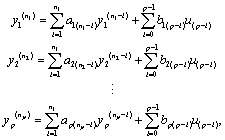(1)

where y is the plant output, and u is the plant input. Plant parameters are defined as a and b. Then, by using unknown internal dynamics f, (1) is rewritten as follows: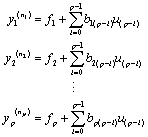(2)

A new state variable is defined by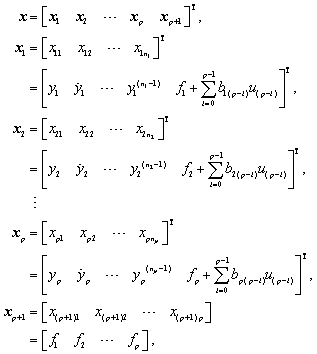(3),

and the manipulated variable is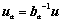(4),

where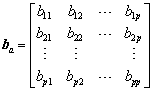Then, the state equation in matrix form is described by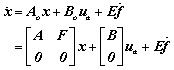(5)

where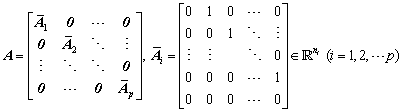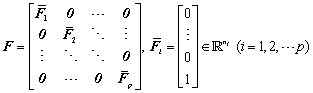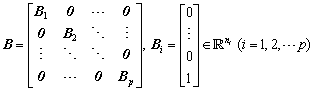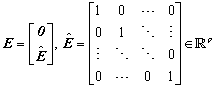The observer for this system is described by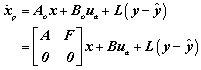(6)

Where f is estimated from the observer. Here L is obtained by the linear–quadratic regulator (LQR). Then, the manipulated variable is given as follows: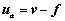(7)

where v is a new manipulated variable. Therefore, (2) is given as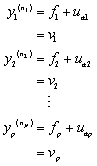(8)

Therefore, the transfer function between y and v is described by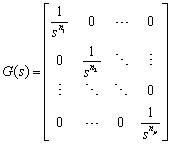(9)

Model following controller

In this section, the design method of the model-following controller for the converted plant by ADRC is explained.

We state the space model to be as follows: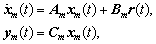(10)

where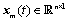is the state vector of the model,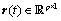is the model input,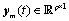is the model output, and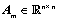,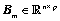, and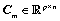are the constant matrices of appropriate dimensions. By denoting the error as en, the following equation can be obtained: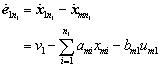(11)

In addition, by denoting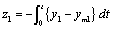, the following equation is obtained :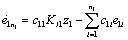(12)

Similarly,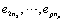are required. Therefore, the manipulated variable is described as: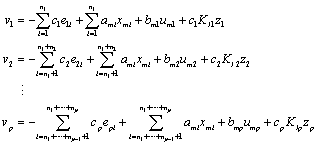(13)

From (11) , (12), and (13), the following equations are derived: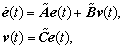(14)

where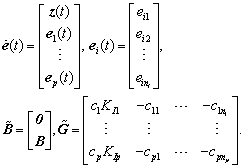The optimal gain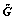is obtained by LQR. Figure 2 shows a block diagram of the two-input two-output system of the proposed method. Here the cases of the orders of the model and the plant being the same and different are considered.

Case 1: Second order plant and second order model

The transfer function between the target value r1 and the plant output y1 is described by the following equation: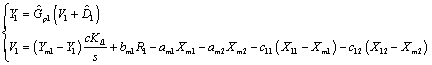(15)

Then, by substituting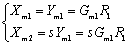(16)

and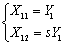(17)

in (15), the following is obtained: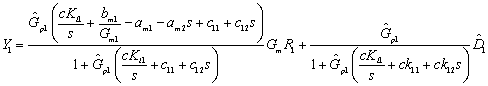(18)

Here by substituting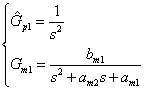(19)

in (18), the target value response in the system is described by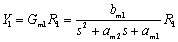(20)

Moreover, disturbance response is described by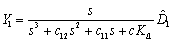(21)

Using the final-value theorem, the system is not affected by disturbance. By similarly calculating the transfer function between ri and yi (i = 2... p) , the whole target value corresponds to the model output.

Case 2: Third order plant and second order model

The manipulated variable v1 is described by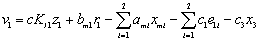(22)

where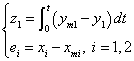(23)

Then, (15) is rewritten as follows: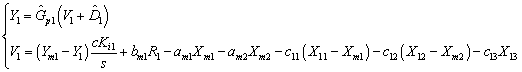(24)

By calculating the response in the same manner as Case 1, the target value response in the system is described by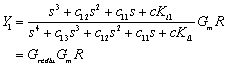(25)

The Bode diagram of Grediu and Gm is shown in Figure 3. Here the parameters that were used in the simulation study are listed in Table 1.

Parameter Value
KI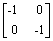C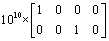L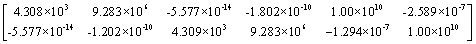Table 1: List of the gain parameters used in section 4.1 and 4.2.

As shown in Figure 3, the cutoff frequency of Grediu was fully larger than the cutoff frequency of Gm. Therefore, the following equation is obtained: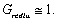(26)

#### Simulation Results

In this section, the effectiveness of the proposed method is shown using the results of a simulation. The control system is simulated using MATLAB/Simulink.

In the case that the order of the plant is equal to the order of the model

An example of the coupled MIMO plant used in this simulation is described as follows: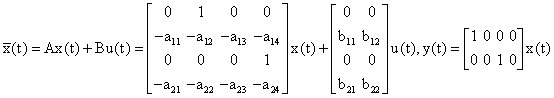, (27)

where: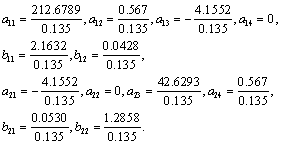The MIMO model is set to: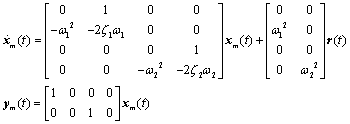(28)

where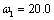,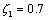,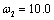, and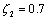. The model input r(t) comprises the step signal and the sine wave.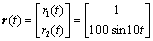. (29)

The gain parameters are listed in Table 1. An input-side step disturbance of magnitude -0.1 was applied at time t=2.0. To show the robustness, the modeling error for the plant was set to be ±50% for the coefficients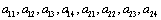. The simulation results for the output responses y1 and y2 of the conventional method  are shown in Figures 4 and 5. The simulation results for the output responses y1 and y2 of the proposed method are shown in Figures 6 and 7.

In the case that the order of the plant is not equal to the order of the model

An example of the coupled MIMO plant of the third-order system used in this simulation is described as follows: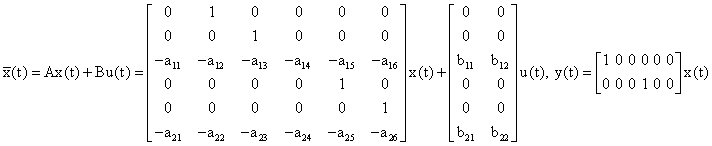(30)

where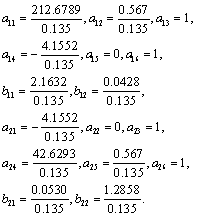The same model and the same model input as those described in Section 3.1 were used. The gain parameters are listed in Table 1. An input-side step disturbance of magnitude -0.1 was applied at time t=2.0. To show the robustness of the method, the modeling error for the plant was set to be ±50% for the coefficients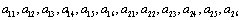. The simulation results for the output responses y1 and y2 of the proposed method are shown in Figures 8 and 9.

Figure 8: Output y1 in the case that the order of the plant is not equal to the order of the model.

Figure 9: Output y2 in the case that the order of the plant is not equal to the order of the model.

#### Experimental Study

In this section, the effectiveness of the proposed method is shown by the experimental results with a MIMO system. A DC motor  was virtually extended from the SISO system to the MIMO system since only SISO equipment was available in the laboratory.

The experimental control system is shown in Figure 10 and comprises a DC motor, an encoder, a counter that measures the information from the encoder, a host PC that transmits the control information, a target PC that calculates the manipulated variable, and a D/A converter.

First, the control signal used to move the DC motor was transmitted from the host PC to the target PC and then to the D/A converter, which had a resolution of 12 bits. Next, the output of the motor was transformed into a pulse of phases A and B by the encoder, which had a resolution of 1.534 × 103 radians/count. The counter measured these pulses and transmitted the positional signal to the host PC through the target PC. In Figure 10 shows the DC motor used in the experimental study, which was made by the PID Corporation (PID-QET ii). The motor was equipped with an inertial load disk with a radius of 0.0248 m and a mass of 0.068 kg. The control plant was identified by the step response method as follows: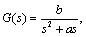(31)

where a =10.78 and b = 339.6 .

Moreover, a DC motor was virtually extended from the SISO system to the MIMO system. As shown in Figure 11, a DC motor was part of the system. Control object 1 is a DC motor, and the transfer function of control object 2 is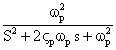(32)

The gain parameters were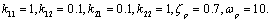(33)

The experimental results for the output response are shown in Figure 12. The effectiveness of the proposed method was confirmed by the experimental results to be similar to those predicted by the simulation.

#### Conclusions

In this paper, we have proposed a model-following control system design method that is independent of the plant parameter MIMO systems. The proposed method can prevent the influence of an input-side disturbance and is robust against parameter changes. Furthermore, it becomes decouplable in the case of MIMO systems. The effectiveness of the proposed method was confirmed by simulation studies in Section 4 and by the experimental results for positioning control with a DC motor in Section 5.

#### References

Select your language of interest to view the total content in your interested language

### Article Usage

• Total views: 9543
• [From(publication date):
March-2016 - Sep 23, 2019]
• Breakdown by view type
• HTML page views : 9327

## Post your commentCan't read the image? click here to refresh
###### Peer Reviewed Journals

Make the best use of Scientific Research and information from our 700 + peer reviewed, Open Access Journals

International Conferences 2019-20

Meet Inspiring Speakers and Experts at our 3000+ Global Annual Meetings

Top